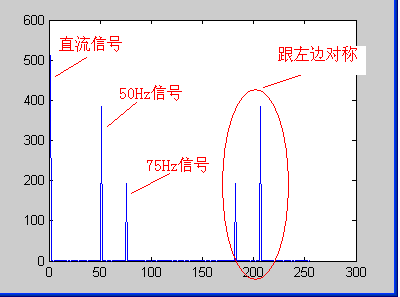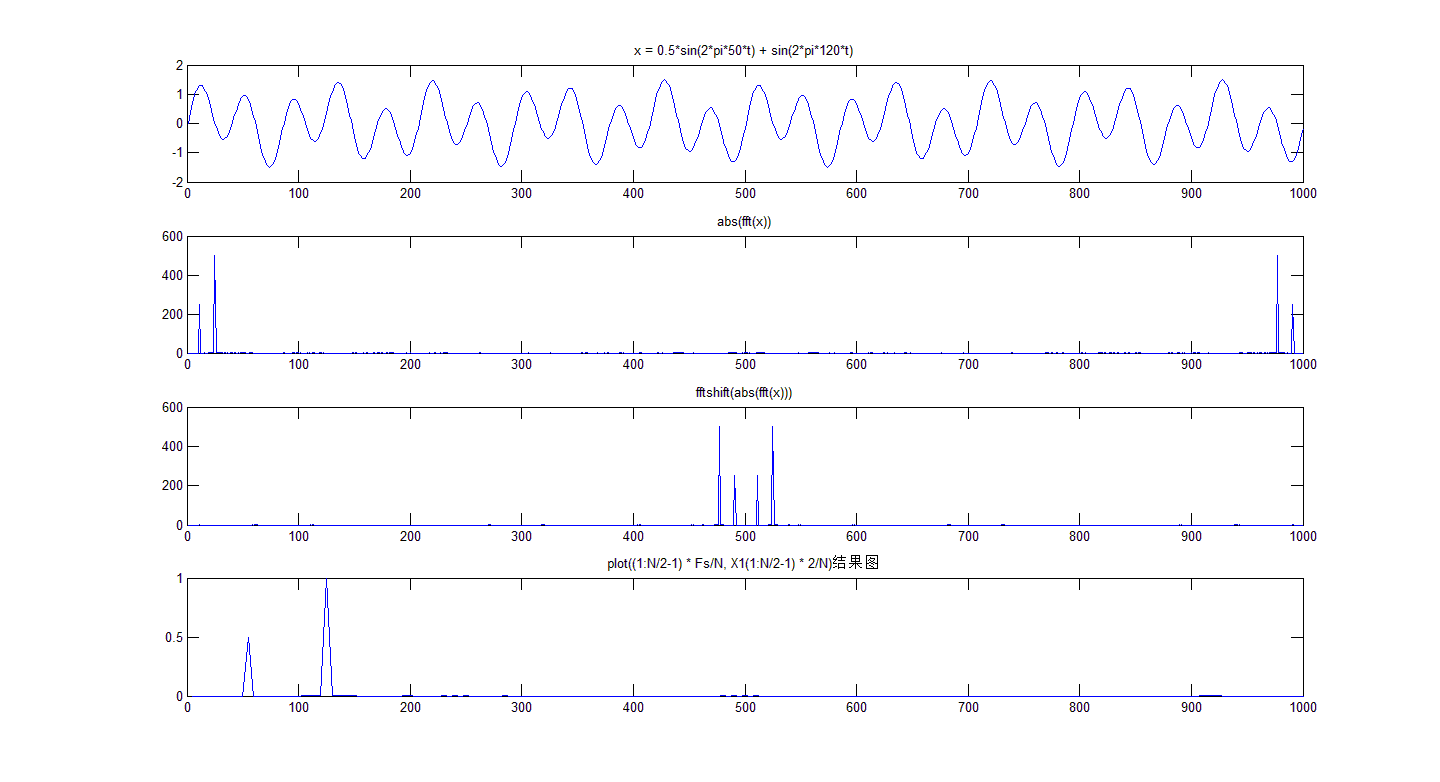• ----------------------------- 更新：添加了易于使用的 GUI ----------------------------- [Px,freq]=... Nfft = FFT 分辨率，默认值为 8192； 采样频率假定为 44.1 kHz。 如果未定义输出，则 averfft 绘制频谱。matlab
• DFT的matlab源代码Goertzel算法和scipy.fftpack.fft的基准 概述 为了评估信号中特定频率分量的功率， Goertzel algorithm将比fast Fourier transform (FFT)是更好的解决方案。 因为Goertzel algorithm允许我们一次...
• STFT（短时傅立叶变换），ISTFT（逆-短时傅立叶变换），用于音频，麦克风输入 提供25％，50％的重叠STFTCraft.io。 笔记 git clone --recursive https://github.com/kooBH/STFT.git 要构建测试代码，您需要克隆--...
• FFT是离散傅立叶变换的快速算法，可以将一个信号变换到频域。... 虽然很多人都知道FFT是什么，可以用来做什么，怎么去做，但是却不知道FFT之后的结果是什意思、如何决定要使用多少点来做FFT。 现在就

学习来源: https://www.cnblogs.com/zhaojihui/p/6684208.html.
FFT是离散傅立叶变换的快速算法，可以将一个信号变换到频域。有些信号在时域上是很难看出什么特征的，但是如果变换到频域之后，就很容易看出特征了。这就是很多信号分析采用FFT变换的原因。另外，FFT可以将一个信号的频谱提取出来，这在频谱分析方面也是经常用的。

虽然很多人都知道FFT是什么，可以用来做什么，怎么去做，但是却不知道FFT之后的结果是什意思、如何决定要使用多少点来做FFT。

采样得到的数字信号，就可以做FFT变换了。N个采样点，经过FFT之后，就可以得到N个点的FFT结果。为了方便进行FFT运算，通常N取2的整数次方。

假设采样频率为Fs，信号频率F，采样点数为N。那么FFT之后结果就是一个为N点的复数。每一个点就对应着一个频率点。这个点的模值，就是该频率值下的幅度特性。具体跟原始信号的幅度有什么关系呢？假设原始信号的峰值为A，那么FFT的结果的每个点（除了第一个点直流分量之外）的模值就是A的N/2倍。而第一个点就是直流分量，它的模值就是直流分量的N倍。而每个点的相位呢，就是在该频率下的信号的相位。第一个点表示直流分量（即0Hz），而最后一个点N的再下一个点（实际上这个点是不存在的，这里是假设的第N+1个点，也可以看做是将第一个点分做两半分，另一半移到最后）则表示采样频率Fs，这中间被N-1个点平均分成N等份，每个点的频率依次增加。例如某点n所表示的频率为：Fn=(n-1)*Fs/N。由上面的公式可以看出，Fn所能分辨到频率为为Fs/N，如果采样频率Fs为1024Hz，采样点数为1024点，则可以分辨到1Hz。1024Hz的采样率采样1024点，刚好是1秒，也就是说，采样1秒时间的信号并做FFT，则结果可以分析到1Hz，如果采样2秒时间的信号并做FFT，则结果可以分析到0.5Hz。如果要提高频率分辨力，则必须增加采样点数，也即采样时间。频率分辨率和采样时间是倒数关系。

假设FFT之后某点n用复数a+bi表示，那么这个复数的模就是An=根号a*a+b*b，相位就是Pn=atan2(b,a)。根据以上的结果，就可以计算出n点（n≠1，且n<=N/2）对应的信号的表达式为：An/(N/2)*cos(2*pi*Fn*t+Pn)，即2*An/N*cos(2*pi*Fn*t+Pn)。对于n=1点的信号，是直流分量，幅度即为A1/N。

由于FFT结果的对称性，通常我们只使用前半部分的结果，即小于采样频率一半的结果。

下面以一个实际的信号来做说明。

假设我们有一个信号，它含有2V的直流分量，频率为50Hz、相位为-30度、幅度为3V的交流信号，以及一个频率为75Hz、相位为90度、幅度为1.5V的交流信号。用数学表达式就是如下：

S=2+3cos(2pi50t-pi30/180)+1.5cos(2pi75t+pi90/180)

式中cos参数为弧度，所以-30度和90度要分别换算成弧度。我们以256Hz的采样率对这个信号进行采样，总共采样256点。按照我们上面的分析，Fn=(n-1)*Fs/N，我们可以知道，每两个点之间的间距就是1Hz，第n个点的频率就是n-1。我们的信号有3个频率：0Hz、50Hz、75Hz，应该分别在第1个点、第51个点、第76个点上出现峰值，其它各点应该接近0。实际情况如何呢？我们来看看FFT的结果的模值如图所示。从图中我们可以看到，在第1点、第51点、和第76点附近有比较大的值。我们分别将这三个点附近的数据拿上来细看：
1点： 512+0i

2点： -2.6195E-14 - 1.4162E-13i

3点： -2.8586E-14 - 1.1898E-13i

50点：-6.2076E-13 - 2.1713E-12i

51点：332.55 - 192i

52点：-1.6707E-12 - 1.5241E-12i

75点：-2.2199E-13 -1.0076E-12i

76点：3.4315E-12 + 192i

77点：-3.0263E-14 +7.5609E-13i
很明显，1点、51点、76点的值都比较大，它附近的点值都很小，可以认为是0，即在那些频率点上的信号幅度为0。接着，我们来计算各点的幅度值。分别计算这三个点的模值，结果如下：

1点： 512

51点：384

76点：192

按照公式，可以计算出直流分量为：512/N=512/256=2；50Hz信号的幅度为：384/(N/2)=384/(256/2)=3；75Hz信号的幅度为192/(N/2)=192/(256/2)=1.5。可见，从频谱分析出来的幅度是正确的。

然后再来计算相位信息。直流信号没有相位可言，不用管它。先计算50Hz信号的相位，atan2(-192, 332.55)=-0.5236,结果是弧度，换算为角度就是180*(-0.5236)/pi=-30.0001。再计算75Hz信号的相位，atan2(192, 3.4315E-12)=1.5708弧度，换算成角度就是180*1.5708/pi=90.0002。可见，相位也是对的。根据FFT结果以及上面的分析计算，我们就可以写出信号的表达式了，它就是我们开始提供的信号。

总结：假设采样频率为Fs，采样点数为N，做FFT之后，某一点n（n从1开始）表示的频率为：Fn=(n-1)*Fs/N；该点的模值除以N/2就是对应该频率下的信号的幅度（对于直流信号是除以N）；该点的相位即是对应该频率下的信号的相位。相位的计算可用函数atan2(b,a)计算。atan2(b,a)是求坐标为(a,b)点的角度值，范围从-pi到pi。要精确到xHz，则需要采样长度为1/x秒的信号，并做FFT。要提高频率分辨率，就需要增加采样点数，这在一些实际的应用中是不现实的，需要在较短的时间内完成分析。解决这个问题的方法有频率细分法，比较简单的方法是采样比较短时间的信号，然后在后面补充一定数量的0，使其长度达到需要的点数，再做FFT，这在一定程度上能够提高频率分辨力。具体的频率细分法可参考相关文献。
[附录：本测试数据使用的matlab程序]

close all; %先关闭所有图片

A1=3; %频率F1信号的幅度

A2=1.5; %频率F2信号的幅度

F1=50; %信号1频率(Hz)

F2=75; %信号2频率(Hz)

Fs=256; %采样频率(Hz)

P1=-30; %信号1相位(度)

P2=90; %信号相位(度)

N=256; %采样点数

t=[0:1/Fs:N/Fs]; %采样时刻

%信号

plot(S);%显示原始信号

title(‘原始信号’);

figure;

Y = fft(S,N); %做FFT变换

Ayy = (abs(Y)); %取模

plot(Ayy(1:N)); %显示原始的FFT模值结果

title(‘FFT 模值’);

figure;

Ayy=Ayy/(N/2); %换算成实际的幅度

Ayy(1)=Ayy(1)/2;

F=([1:N]-1)*Fs/N; %换算成实际的频率值

plot(F(1:N/2),Ayy(1:N/2)); %显示换算后的FFT模值结果

title(‘幅度-频率曲线图’);

figure;

Pyy=[1:N/2];

for i=“1:N/2”

Pyy(i)=phase(Y(i)); %计算相位

Pyy(i)=Pyy(i)*180/pi; %换算为角度

end;

plot(F(1:N/2),Pyy(1:N/2)); %显示相位图

title(‘相位-频率曲线图’)

展开全文matlab fft
• MATLAB中通过fft计算信号频谱的问题

万次阅读 多人点赞 2017-02-17 17:43:08
之前一直在做声音相关的一个...众所周知，fft是快速傅里叶变换，当信号为2的整数幂效率最高（当然还有基为3，4的fft，用的不多此处不表，下面提到的fft均为基是2的fft）。 而现实生活中的信号往往并不是2的整数幂

之前一直在做声音相关的一个项目，其中用到了很多信号频谱的问题，包括fft点数的选取、fft之后画图横纵坐标的问题、fftshift的用法等等。前面因为忙，也没有仔细研究，现在将问题总结如下：

1.fft点数的选取。

众所周知，fft是快速傅里叶变换，当信号为2的整数幂时效率最高（当然还有基为3，4的fft，用的不多此处不表，下面提到的fft均为基是2的fft）。
而现实生活中的信号往往并不是2的整数幂，那么这时候应该怎么办呢？
个人认为解决方案有两种：
（1）采用离散傅里叶变换（DFT）的定义来做，这样就能保证对任意点数都能处理，但缺点就是计算量大，速度太慢。因此当数据量小的时候，可以这么用。
（2）在原始信号后面补零，使其长度变成2的整数幂。这是目前最常见的做法，matlab里面的fft函数就是这样做的。好处是有很多现成的fft算法，当你信号长度2的整数幂时，速度会很快、很方便，缺点则是精度上有点差距。

2.fft之后画图横纵坐标的问题以及fftshift的用法。

之前用matlab中的fft，只是用用函数，并未对其进行全面了解，但最近在画频谱图的时候发现横纵坐标的意义根本不懂，fftshift又是怎么用呢？下面通过一段代码和实验说明。

Fs = 5000; % Sampling frequency
T = 1/Fs; % Sample time
N = 1000; % Length of signal
t = (0:N-1)/Fs; % Time vector

x = 0.5*sin(2*pi*50*t) + sin(2*pi*120*t);
X1=abs(fft(x))

xx1=abs(fft(x));
xx2=fftshift(abs(fft(x)));

figure
subplot(4,1,1)
plot(x);
title(‘x = 0.5*sin(2*pi*50*t) + sin(2*pi*120*t)’);
subplot(4,1,2)
plot(xx1);
title(‘abs(fft(x))’);
subplot(4,1,3)
plot(xx2);
title(‘fftshift(abs(fft(x)))’);
subplot(4,1,4)
plot((1:N/2-1)*Fs/N,X1(1:N/2-1))*2/N;;
title(‘plot((1:N/2-1) * Fs/N, X1(1:N/2-1) * 2/N)结果图’);可以看出，
（1）经过matlab里abs(fft(x))的结果即为信号x的频谱，而加上fftshift则是将信号关于0频进行对称。
（2）abs(fft(x))取前半部分，fftshift(abs(fft(x)))取后半部分，则为正确的频谱图（但横纵坐标不对）。
（3）最终正确的频谱图为第四个子图所示，横纵坐标均需要特殊处理：
纵坐标： abs(fft(x))* 2 / N才为真实频谱幅值。
横坐标： plot((1:N/2-1) * Fs/N, X1(1:N/2-1) )，只取一半，并且要乘以频率分辨率Fs/N才为真实的频率值。

展开全文matlab fft fft横坐标 频谱图 频率分辨率
• 短时频谱分析,频谱图的伪彩色映射及显示.频谱图的类型有宽带和窄带两种,伪彩色显示的映射 可按需求设置.文中介绍了基于FFT频谱分析与频谱图显示的原理,Matlab的相关功能函数,并 给出了一个程序实例及其实验结果....matlab
• MATLAB实现短时傅里叶变换

千次阅读 2020-10-19 15:54:37
一、短时傅里叶变换的定义 离散傅里叶变换使用的是一种全局变换，因为它表示一段时间内平均的频率特性，无法表述信号的时域局域性质，为了能够分析处理非平稳信号，人们对离散傅里叶变换进行了推广，提出了短时...

一、短时傅里叶变换的定义

离散傅里叶变换使用的是一种全局变换，因为它表示一段时间内平均的频率特性，无法表述信号的时域局域性质，为了能够分析处理非平稳信号，人们对离散傅里叶变换进行了推广，提出了短时傅里叶变换。表达式如下所示：短时傅立叶采用滑动窗口机制，设定窗口大小和步长，让窗口在时域信号上滑动，分别计算每个窗口的傅立叶变换形成了不同时间窗口对应的频域信号，拼接起来就成为了频率随时间变化的数据（时频信号）。二、函数调用

1.短时傅里叶变换tfrstft函数：

[tfr,t,f]=tfrstft(x,t,N,h，trace);

其中x是数据矢量，t是时间刻度（默认1：N），N是FFT长度，h是窗函数，trace为是否跟踪运算。tfr是x的STFT值，t是时间刻度的输出变量，f是频率刻度的输出变量，是一个归一化的频率值，在-0.5-0.5的区间内。

2.短时傅里叶逆变换tfristft

[x,t]=tfristft(tfr,t,N,h，trace);

tfr是STFT域的数值，是一个二维的复数数组，t是时间刻度，x是经短时傅里叶逆变换得到的重构数据，t是该重构数据对应的时间刻度。

三、用tfrstft函数做STFT的谱图

1.mesh函数作三维图：

mesh(t,f(1:N/2)*fs,abs(tfr(1:N/2,:)));2.mesh函数做二维图：

mesh(tt,f(1:N/2)*fs,abs(B(1:N/2,:)));
view(0,90); xlim([0 max(tt)])%使用view函数翻转mesh所做的三维图3.imagesc函数做二维图

imagesc(tt,f(1:N/2)*fs,abs(B(1:N/2,:))); axis xy

附：
（1）imagesc函数用法：
imagesc函数用法
（2）axis xy：
axis xy与axis ij的用法4.范例

clear all; clc; close all;
N=1024;                      % 数据长度
fs=1000;                     % 采样频率
tt=(0:N-1)/fs;               % 时间刻度
x1=chirp(tt,0,1,350);        % Chirp 信号x1
x2=chirp(tt,350,1,0);        % Chirp 信号x2
x=x1'+x2';                   % 两个Chirp 信号相加；
win=hanning(127);            % 窗函数
[B,t,f]=tfrstft(x,1:N,N,win);% 短时傅里叶变换
% 作图
figure(1)                    % 信号波形图
subplot 211; plot(tt,x1,'k');
title(' Chirp信号x1')
xlabel('时间/s'); ylabel('幅值')
xlim([0 max(tt)]);
subplot 212; plot(tt,x2,'k');
title(' Chirp信号x2')
xlabel('时间/s'); ylabel('幅值')
xlim([0 max(tt)]);
set(gcf,'color','w');
figure(2)                    % 用mesh作三维图
mesh(tt,f(1:N/2)*fs,abs(B(1:N/2,:)));
xlabel('时间/s'); ylabel('频率')
title('调频信号STFT的三维图')
axis([0 max(tt) 0 500 0 6]);
set(gcf,'color','w');
figure(3)                    % 用mesh作二维图
mesh(tt,f(1:N/2)*fs,abs(B(1:N/2,:)));
view(0,90); xlim([0 max(tt)])
xlabel('时间/s'); ylabel('频率')
title('调频信号STFT的二维图')
set(gcf,'color','w');
figure(4)                    % 用contour作等高线图
contour(tt,2*f(1:N/2)*fs,abs(B(1:N/2,:)),256);
xlabel('时间/s'); ylabel('频率')
title('调频信号STFT的等高线图')
set(gcf,'color','w');
figure(5)                    % 用imagesc作二维图
imagesc(tt,f(1:N/2)*fs,abs(B(1:N/2,:)));
axis xy
xlabel('时间/s'); ylabel('频率')
title('调频信号STFT的频谱图')
set(gcf,'color','w');

注意：
（1）调用tfrstft函数时信号数据必须为一个列矢量
（2）FFT点数必须是2的整数次幂
（3）输出矩阵B是一个复数矩阵，在看STFT频谱图时，通常观察幅值谱，所以在作图时使用abs（B），注意设置正品率N/2
（4）tfr函数的输出t是0：N-1而不是实际时间刻度。
（5）tfr函数的输出f是归一化频率，不是实际频率
（6）在绘图时，要对频率轴和时间轴进行修正。时间轴要设置为**（0：N-1）/fs**，频率轴要设置为2ffs

四、窗的长度对短时傅里叶变换的影响

1.代码：

clear all; clc; close all;
fs=1000;                     % 采样频率
tt=(0:1000)'/fs;             % 时间刻度
% 构成信号
x=sin(2*pi*400*tt).*(tt<=0.3)+sin(2*pi*200*tt).*(tt>0.3&tt<=0.6)+...
sin(2*pi*100*tt).*(tt>0.6&tt<=0.8)+sin(2*pi*50*tt).*(tt>0.8);

N=length(x);                 % 数据长度
nfft=256;                    % 设置nfft
n3=1:128;                    % 设置正频率部分
h=hamming(31);                     % 设置窗长为31
[tfr1,t,f1]=tfrstft(x,1:N,nfft,h); % STFT
h=hamming(63);                     % 设置窗长为63
[tfr2,t,f2]=tfrstft(x,1:N,nfft,h); % STFT
h=hamming(127);                    % 设置窗长为127
[tfr3,t,f3]=tfrstft(x,1:N,nfft,h); % STFT
h=hamming(255);                    % 设置窗长为255
[tfr4,t,f4]=tfrstft(x,1:N,nfft,h); % STFT
% 作图
figure(1)
pos = get(gcf,'Position');
set(gcf,'Position',[pos(1), pos(2)-100,pos(3),(pos(4)-180)]);
plot(tt,x,'k');
xlabel('时间/s'); ylabel('幅值'); title('信号波形图')
set(gcf,'color','w');
figure(2)
subplot 221; imagesc(tt,2*f1(n3)*fs,abs(tfr1(n3,:)));
set(gca, 'YTickmode', 'manual', 'YTick', [0,50,100,200,400,500]);
grid; title('窗长=31'); ylabel('频率/Hz'); xlabel('时间/s');
subplot 222; imagesc(tt,2*f2(n3)*fs,abs(tfr2(n3,:)));
set(gca, 'YTickmode', 'manual', 'YTick', [0,50,100,200,400,500]);
grid; title('窗长=63'); ylabel('频率/Hz'); xlabel('时间/s');
subplot 223; imagesc(tt,2*f3(n3)*fs,abs(tfr3(n3,:)));
set(gca, 'YTickmode', 'manual', 'YTick', [0,50,100,200,400,500]);
grid; title('窗长=127'); ylabel('频率/Hz'); xlabel('时间/s');
subplot 224; imagesc(tt,2*f4(n3)*fs,abs(tfr4(n3,:)));
set(gca, 'YTickmode', 'manual', 'YTick', [0,50,100,200,400,500]);
grid; title('窗长=255'); ylabel('频率/Hz'); xlabel('时间/s');
set(gcf,'color','w');

2.结果分析：可以看到当窗函数窗长较短时，信号谱图中的频带变粗，与普通FFT变换结果一样，虽然都是按照256点进行FFT分析，但不同窗长有效数据的长度是不同的，窗长短的频率分辨率低。但也不一定窗长越长越好，可以看到窗长为255的时候，出现了200和400频率相重叠的情况。因此在进行STFT分析时既要考虑频率的分辨率，也要考虑防止在时间长的混叠，取一个折中的窗长。

1.代码：

clear all; clc; close all;
N=length(x);                           % 信号长度
tt=(0:N-1)/fs;                         % 时间刻度
h=hanning(63);                         % 窗长63
[B1,t,f1]=tfrstft(x,1:N,64,h);         % Nfft=64
[B2,t,f2]=tfrstft(x,1:N,1024,h);       % Nfft=1024
% 作图r
subplot 211; mesh(tt,2*f1(1:32)*fs,abs(B1(1:32,:))); axis xy
xlabel('时间/s'); ylabel('频率/Hz');
title('Nfft=64');
subplot 212; mesh(tt,2*f2(1:512)*fs,abs(B2(1:512,:))); axis xy
xlabel('时间/s'); ylabel('频率/Hz');
title('Nfft=1024');
set(gcf,'color','w');

2.结果对比：可以看到nfft值较小时，得到的语音谐波结构不如nfft值较大时光滑。所以在相同窗长时也要选择合适的nfft值。

语音信号的分帧通过加权窗滑动得到？

展开全文• 利用matlab怎样进行频谱分析_大漠东风_新浪博客 MATLAB怎样实现FFTMatlab实现快速傅立叶变换 FFT是离散傅立叶变换的快速算法，可以将一个信号变换到频域。有些信号在时域上是很难看出什么特征的，但是如果变换到...

Version:1.0 StartHTML:000000215 EndHTML:000069168 StartFragment:000005330 EndFragment:000069076 StartSelection:000006409 EndSelection:000069072 SourceURL:http://blog.sina.com.cn/s/blog_4405fa340102xjym.html[转载]利用matlab怎样进行频谱分析_大漠东风_新浪博客

MATLAB怎样实现FFT

Matlab实现快速傅立叶变换

FFT是离散傅立叶变换的快速算法，可以将一个信号变换到频域。有些信号在时域上是很难看出什么特征的，但是如果变换到频域之后，就很容易看出特征了。这就是很多信号分析采用FFT变换的原因。另外，FFT可以将一个信号的频谱提取出来，这在频谱分析方面也是经常用的。
虽然很多人都知道FFT是什么，可以用来做什么，怎么去做，但是却不知道FFT之后的结果是什意思、如何决定要使用多少点来做FFT
采样得到的数字信号，就可以做FFT变换了。N个采样点，经过FFT之后，就可以得到N个点的FFT结果。为了方便进行FFT运算，通常N2的整数次方。
假设采样频率为Fs，信号频率F，采样点数为N。那么FFT之后结果就是一个为N点的复数。每一个点就对应着一个频率点。这个点的模值，就是该频率值下的幅度特性。具体跟原始信号的幅度有什么关系呢？假设原始信号的峰值为A，那么FFT的结果的每个点（除了第一个点直流分量之外）的模值就是AN/2。而第一个点就是直流分量，它的模值就是直流分量的N而每个点的相位呢，就是在该频率下的信号的相位。第一个点表示直流分量（即0Hz），而最后一个点N的再下一个点（实际上这个点是不存在的，这里是假设的第N+1个点，也可以看做是将第一个点分做两半分，另一半移到最后）则表示采样频率Fs，这中间被N-1个点平均分成N等份，每个点的频率依次增加。例如某点n所表示的频率为：Fn=(n-1)*Fs/N。由上面的公式可以看出，Fn所能分辨到频率为Fs/N，如果采样频率Fs1024Hz，采样点数为1024点，则可以分辨到1Hz1024Hz的采样率采样1024点，刚好是1秒，也就是说，采样1秒时间的信号并做FFT，则结果可以分析到1Hz如果采样2秒时间的信号并做FFT，（
是否应该说采样点数为2*1024,并不是2s的数据））则结果可以分析到0.5Hz如果要提高频率分辨力，则必须增加采样点数，也即采样时间。频率分辨率和采样时间是倒数关系。
假设FFT之后某点n用复数a+bi表示，那么这个复数的模就是An=根号a*a+b*b，相位就是Pn=atan2(b,a)。根据以上的结果，就可以计算出n点（n≠1，且n<=N/2）对应的信号的表达式为：An/(N/2)*cos(2*pi*Fn*t+Pn)，即2*An/N*cos(2*pi*Fn*t+Pn)。对于n=1点的信号，是直流分量，幅度即为A1/N由于FFT结果的对称性，通常我们只使用前半部分的结果，即小于采样频率一半的结果。
下面以一个实际的信号来做说明。假设我们有一个信号，它含有2V的直流分量，频率为50Hz、相位为-30度、幅度为3V的交流信号，以及一个频率（f0）为75Hz、相位为90度、幅度为1.5V的交流信号。用数学表达式就是如下：S=2+3*cos(2*pi*50*t-pi*30/180)+1.5*cos(2*pi*75*t+pi*90/180)。式中cos参数为弧度，所以-30度和90度要分别换算成弧度。我们以256Hz的采样率对这个信号进行采样，总共采样256点。按照我们上面的分析，Fn=(n-1)*Fs/N，我们可以知道，每两个点之间的间距就是1Hz，第n个点的频率就是n-1。我们的信号有3个频率：0Hz50Hz75Hz，应该分别在第1个点、第51个点、第76个点上出现峰值，其它各点应该接近0。实际情况如何呢？我们来看看FFT的结果的模值如图所示。

从图中我们可以看到，在第1点、第51点、和第76点附近有比较大的值。我们分别将这三个点附近的数据拿上来细看：
1点： 512+0i
2点： -2.6195E-14 - 1.4162E-13i
3点： -2.8586E-14 - 1.1898E-13i
50点：-6.2076E-13 - 2.1713E-12i
51点：332.55 - 192i
52点：-1.6707E-12 - 1.5241E-12i
75点：-2.2199E-13 -1.0076E-12i
76点：3.4315E-12 + 192i
77点：-3.0263E-14 +7.5609E-13i
很明显，1点、51点、76点的值都比较大，它附近的点值都很小，可以认为是0，即在那些频率点上的信号幅度为0。接着，我们来计算各点的幅度值。分别计算这三个点的模值，结果如下：
1点： 512
51点：384
76点：192
按照公式，可以计算出直流分量为：512/N=512/256=250Hz信号的幅度为：384/(N/2)=384/(256/2)=375Hz信号的幅度为192/(N/2)=192/(256/2)=1.5。可见，从频谱分析出来的幅度是正确的。
然后再来计算相位信息。直流信号没有相位可言，不用管它。先计算50Hz信号的相位，atan2(-192, 332.55)=-0.5236,结果是弧度，换算为角度就是180*(-0.5236)/pi=-30.0001。再计算75Hz信号的相位，atan2(192, 3.4315E-12)=1.5708弧度，换算成角度就是180*1.5708/pi=90.0002。可见，相位也是对的。根据FFT结果以及上面的分析计算，我们就可以写出信号的表达式了，它就是我们开始提供的信号。

总结：假设采样频率为Fs，采样点数为N，做FFT之后，某一点nn1开始）表示的频率为：Fn=(n-1)*Fs/N；该点的模值除以N/2就是对应该频率下的信号的幅度（对于直流信号是除以N）；该点的相位即是对应该频率下的信号的相位。相位的计算可用函数atan2(b,a)计算。atan2(b,a)是求坐标为(a,b)点的角度值，范围从-pipi。要精确到xHz，则需要采样长度为1/x秒的信号，并做FFT。要提高频率分辨率，就需要增加采样点数，这在一些实际的应用中是不现实的，需要在较短的时间内完成分析。解决这个问题的方法有频率细分法，比较简单的方法是采样比较短时间的信号，然后在后面补充一定数量的0，使其长度达到需要的点数，再做FFT，这在一定程度上能够提高频率分辨力。具体的频率细分法可参考相关文献。

附贴上上述例子的matlab程序：

Matlab的例子（一）

t=0:1/256:1;%采样步长

y= 2+3*cos(2*pi*50*t-pi*30/180)+1.5*cos(2*pi*75*t+pi*90/180);

N=length(t); %样点个数

plot(t,y);

fs=256;%采样频率

df=fs/(N-1) ;%分辨率

f=(0:N-1)*df;%其中每点的频率

Y=fft(y)/N*2;%真实的幅值

%Y=fftshift(Y);

figure(2)

plot(f,abs(Y));

由于以上程序是结合傅里叶算法转换得到的对称图，而常用的只需要一半就可以了。对应的程序如下：

t=0:1/256:1;%采样步长

y= 2+3*cos(2*pi*50*t-pi*30/180)+1.5*cos(2*pi*75*t+pi*90/180);

N=length(t); %样点个数

plot(t,y);

fs=256;%采样频率

df=fs/(N-1);%分辨率

f=(0:N-1)*df;%其中每点的频率

Y=fft(y(1:N))/N*2;%真实的幅值

%Y=fftshift(Y);

figure(2)

plot(f(1:N/2),abs(Y(1:N/2)));

附一个计算fft的文件，MATLAB里面计算，输入数组和点数，已验证有效（数组是列还是行，可能需要自己调）

若将原始数据继续扩大N倍，fft的结果也会扩大N倍； 若查表数据扩大N倍，在使用到查表对应的值时，待处理结束后除以N即可，但是会引入误差；

function result = FFT_RIGHT(x, N)

x = double(x);
f = 1;
writetex = 0;
if writetex == 1;
fid = fopen('.\fft_vivien.txt','w'); %获取目标文件写入权限
end

M = log2(N);
if length(x) < N ;
x = [x zeros(1,N-length(x))];                   %若x的长度不是2的整数次幂，则补零至N
end
BRTable = bin2dec(fliplr(dec2bin([1:N]-1,M))) + 1;  %求 1：N 序列序号的倒位序:1.将系数取二进制并对调,然后取十进制
%2.matlab的下标从1开始，需要加1
Xreal = x(BRTable);                                 %调整x输入顺序后的序列，并作为X的初始化
Ximag = zeros(1,N);
X = x(BRTable);
WN = exp(-j*2*pi/N);
for L = 1:M;
B = 2^(L-1);                                    %第L级中，每个蝶形的两个输入数据相聚B个点，共有B个旋转因子
for J = 0:B-1;                                  %第L级中不同的旋转因子
p = J*2^(M-L);                              %旋转因子的指数
WNp = WN^p;
%p = p*j;
for k = J+1: 2^L : N;
%计算出来的包括实部和虚部  此种方法已验证正确
f=0;
if f>0
t = X(k+B) * WNp;                         %产生错误：未定义与'cell'类型的输入参数相对应的运算符‘*’-x=[]非x={}
X(k+B) = X(k)-t;                          %将公式写成指数的形式，按虚实区分
X(k) = X(k)+t;
else

Wncosp = cos(2*pi/N*p);
Wnsinp = sin(2*pi/N*p);
Treal = Xreal(k+B)*Wncosp+Ximag(k+B)*Wnsinp;
Timag = Ximag(k+B)*Wncosp-Xreal(k+B)*Wnsinp;
Xreal(k+B) = Xreal(k) -Treal;
Ximag(k+B) = Ximag(k) -Timag;
Xreal(k) = Xreal(k)+Treal;
Ximag(k) = Ximag(k)+Timag;
end
end
end
end

%求数据的模
for i = 1:1:N;
Rereal(i) = Xreal(i)*Xreal(i);
Reimag(i) = Ximag(i)*Ximag(i);
Result(i) = sqrt(Rereal(i)+Reimag(i));
fprintf('%d \r\n ',Result(i));
if writetex == 1  %写入操作
fprintf(fid,'%d, %d,%d, %d \n',i,Rereal(i),Reimag(i),Result(i) ); %将数据写入目标文件
end
end

if writetex == 1
fclose(fid); %关闭对目标文件操作的入口
end

end

展开全文• 支持FFT点数调整 支持STFT步进调整 支持窗含数据类型调整 支持数据格式调整double、single %%对照组，直接FFT Wave_Length=10240; PointNum=256; Steps=64; Times=(Wave_Length-PointNum)/Steps+1; Waveform_Step=...matlab stft 数字信号处理
• 快速矩形短时傅立叶变换（Cooley-Tukey FFT）的Python实现 频率呈线性增加的信号 窄窗 宽窗 频率呈二次递增的信号 窄窗 宽窗
• 此实时脚本显示了如何处理具有奇数和偶数长度 FFT 的信号。 计算幅度、相位和相应的频率矢量。... 应用包括信号的频率分析、短时傅立叶变换 (STFT)、理想滤波（与对称 ifft 结合），以及其他一些应用。matlab
• FFT算法实现与分析MATLAB

千次阅读 2020-11-28 09:47:33
IV、熟悉MatLab编程。 2.2实验原理 一个连续信号Xa(t)的频谱可以用它的傅里叶变换表示为： 如果对该信号进行理想采样，可以得到采样序列： 同样可以对该序列进行z变换，其中T为采样周期： 当z=e^jω的时候，我们...数字信号处理 matlab fft
• Matlab中FFT与Python的不同结果

千次阅读 2021-02-03 19:08:10
这是用FFT完成的，但是Matlab和Python中的两种方法给出了不同的结果。在Matlab代码：nsamples = length(acc(:,1));domega = 2*pi/(dt*nsamples);acchat = fft(acc);% Make frequency array:Omega = zeros(nsamples,1...
• maxwell 电机气隙磁密与用 matlab 进行 fft 谐波分析 1.对电...matlab谐波分析程序_数学_自然科学_专业资料。clc clear all;.... 用 MATLAB 进行 FFT 频谱分析假设一信号: R 0.6 0.1sin2t / 2.996 0.1cos2t / 7.92 2...
• 数字信号处理（DSP：Digital Signal Process）是电子通信领域非常重要的研究方向，博主汇总了数字信号处理（DSP）中常用的经典案例分析，主要基于算法分析、MATLAB程序实现、信号图像显示，对数字信号处理的实际应用...matlab 算法 DSP
• 短时傅里叶变换（stft）的Matlab源码，已封装为Function函数，可直接根据源码注释调用
• 文章目录Matlab FFT 时域、频域分析ARM Matlab FFT 时域、频域分析 FFT是离散傅立叶变换的快速算法，可以将一个信号变换到频域。有些信号在时域上是很难看出什么特征的，但是如果变换到频域之后，就很容易看出特征了...matlab arm c语言
• matlab时频分析之短时傅里叶变换 spectrogram

万次阅读 多人点赞 2019-03-22 15:50:25
matlab时频分析之短时傅里叶变换 spectrogram 短时傅里叶变换常用于缓慢时变信号的频谱分析，可以观察沿时间变化的频谱信号。 其优点如下图所示，弥补了频谱分析中不能观察时间的缺点，也弥补了时域分析不能获取频率...matlab 短时傅里叶变换 STFT 时频分析 信号处理
• 探秘MATLAB求FFT，计算能量谱

万次阅读 2017-01-17 14:44:44
FFT是离散傅立叶变换的快速算法，虽然很多人都知道FFT是什么，可以用来做什么，怎么去做，但是却不知道FFT之后的结果是什意思、如何决定要使用多少点来做FFT。现在说说FFT结果的具体物理意义。matlab fft 能量谱
•   在Matlab中，做短时傅里叶变换需要使用函数spectrogram，而在Matlab2019中，引入了一个新的函数stft，下面我们就来看下这两个函数都如何使用。   短时傅里叶变换的基本原理就是将数据分段加窗，做fft，在分段...Matlab 短时傅立叶变换 stft 信号处理
• 双踪示波器 1台3．MCOM－TG305数字信号处理与现代通信技术实验箱 1台4．PC机(装有MATLAB、MCOM－TG305配套实验软件) 1台1.3 实验原理一个典型的DSP系统除了数字信号处理部分外，还包括A/D和D/A两部分。这是因为自然...
• v1.0 可编辑可修改 按时间抽取的基 2FFT算法分析及 MATLAB实现 一 DIT-FFT 算法的基本原理 基 2FFT算法的基本思想是把原始的 N点序列依次分解成一系列序列充分利用旋转因子 的周期性和对称性分别求出这些序列...
• 短时傅里叶变换的matlab实现，有详尽的注释，方便学习理解
• matlab的fft函数

2021-04-21 20:36:36
matlabfft的用法及注意事项_调查/报告_表格/模板_实用文档。本文是笔者整理的如何使用matlabfft函数及fftshift函数,希望对大家有所帮助!...C 语言、MATLAB 实现 FFT 几种方法 总结前人经验,仅供参考 ///一...用...
• 解析MATLAB短时傅里叶变换函数spectrogram()

万次阅读 多人点赞 2020-03-15 15:13:28
BB： 最近做脑电信号的时频...Abstract: 我想这篇博文可以帮你弄清楚这几个问题，spectrogram()函数有无返回值调用图像如何转换，函数输入输出参数的的含义，以及其对谱图分辨力的影响。 Note: spectrogram()函数...matlab 信号处理 stft
• 短时傅里叶变换原理及其MATLAB实现（Short Time Fourier Transform，STFT） 1.短时Fourier变换原理（STFT原理） 信号x(t)短时Fourier变换定义为： 其中w(τ)为窗函数。 X(ω,t)中的时间t表示窗函数w(τ−t)的位置，...
• 给出了完整的FFT的C语言代码，以及MATLAB的仿真效果，主要解决了FFT计算频谱图的问题。注意大部分周期信号的最小非零频率分量就是基波，但是还有很多信号并不是这样：比如y=sin(2πx) +sin(3πx)的信号周期为2，对应...
• 引用：http://publishblog.blogchina.com/blog/tb.b?diaryID=3811777在DSP运算中，经常需要把输入时域信号在频域进行... FFT -> X(f) -> 频域处理 -> Y(f) -> IFFT -> y(n)而一般的DSP芯片只支持整数......

matlab短时fftmatlab 订阅